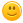krasi_stef 4 Точки

## 07. Name Wars

Molia za pomoshtfunction nameWars(input) {

let name = input.shift();

let current = 0;

let max = 0;

let namemax = "";

let max1 = Number.MIN_SAFE_INTEGER;

while(true){

if (name == "STOP") {

break;

for (let i = 0; i < name.length; i++) {

current = current + name.charCodeAt(i);

if (current > max1 && current>max){

max = current;

namemax = name;

current = 0;

}

}

}

console.log(`Winner is \${namemax} - \${max}!`);

}

}

Тагове:
0chrisi2712 271 Точки

Вашият код 100 от 100. Имахте грешка на ред 7ми, там стрингът трябва да е празен.

function nameWars(input) {

let name = input.shift();

let maxSum = 0;

let greatestName = "";

while(name!="STOP"){

let sum = 0;

for(let i = 0; i < name.length; i++){

sum += name.charCodeAt(i);

}

if (sum > maxSum){

maxSum = sum;

greatestName = name;

}

name = input.shift();

}

console.log(`Winner is \${greatestName} - \${maxSum}!`);

}

0LeonLalli 0 Точки

The people who belong from IT can help you out. Only I can say here that your blog is very interesting and it has wonderful technical information as compared to other sites. I have seen in their top essay writing services work there is no clarity, you explain so well.

0